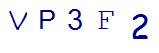# What to Expect with 8th Grade Math ProblemsLast Updated on August 3, 2023 by user

When your student starts 8th grade math problems, you may suddenly feel like math class has gone beyond your ability to help. While recent core standards have changed the face of eighth grade math class, and you may not recognize some of the work coming home, there are resources you can use to provide the assistance your child needs. Sometimes, knowing what to expect can help. Here are some common questions you can expect to see on your child’s 8th grade math problems.

## Linear Equations

8th grade math problems begin to introduce linear equations and systems of linear equations, which will be an important concept in later algebra classes. You can expect to see problems in the form of y = mx + b, and students should be able to know how to graph lines in this format, plotting coordinates using the slope (m) and y-intercept (b).

Here’s how this works. If the student sees y = 2/5x + 5, he is going to begin this graph by creating an x, y coordinate plane. The vertical line on the plane, the y-axis, is where graphing begins.

The student should then place a point at the y-intercept (5). From there, the student measures the slope, which is shown as a ratio of rise/run (familiar?). In this equation, the slope is 2/5. From the 5, the student should go up (rise) 2 and over (run) 5 on the coordinate plane, plotting a second point. Connect the points and extend the line to graph the equation.

Students will also be taught how to solve for one variable in a linear equation. For example, if they are given 3x + 1 = 7, they will know how to solve to get the answer x = 2.

## Functions

In 8th grade, students will begin working with functions. Functions define an output that applies to every number placed into the function. For example, you may see:

f(x) = x+1

This function means that for any number (x) placed into the function, you will add one. So, if the student is asked to find the output or value of the function for 2, 4 and 6, the answer will be 3, 5 and 7 after each value is placed into the function.

## Exponents and Roots

In 8th grade, your student will start working with exponents, square roots and cubed roots. This will also bring in a discussion of irrational numbers. For example, students should be able to identify that the square root of 2 is irrational. In this discussion, you will also see some teaching and questioning about scientific notation. Scientific notation involves writing numbers as multiples of 10 to a certain power. In scientific notation, 2,400 becomes 2.4 x 103.

## Distance and Angles

Another important math concept tackled in 8th grade is the idea of distance and angles. Students will go beyond simply identifying and measuring angles, and will begin to determine how angles will behave when rotated, reflected, dilated and translated. They will also learn ideas about congruence and similarity. When working with distance, students will be able to apply the Pythagorean Theorem (a2 + b2 = c2) and its converse to find distances between points on the coordinate plane.

## Do these concepts seem overwhelming?

If your student needs help, consider investing in Thinkster, an online math tutoring program that provides instruction for students at the point of learning. Created with the help of qualified teachers, this program will give your child the tools and confidence needed to excel with 8th grade math problems.SummaryArticle Name
What to Expect with 8th Grade Math Problems
Description
If your child is about to start 8th grade, you may notice a huge change in the difficulty of their math lessons. So be prepared for 8th grade math problems.
Author
Publisher Name
Thinkster Math
Publisher Logo

## Subscribe to Thinkster Blog## Recommended Articles### Unlocking the Code: Teaching Mathematical Symbols and Their Meaning

Knowledge of mathematical symbols is a foundational skill that empowers students to engage with and excel i...